﻿ Black Hole Entropy, Verlinde’s Entropy Gravity Proposal and Unruh Formula in Projection GravitationPublications are Open
Access in this journal
Article Versions
Export Article
• Normal Style
• MLA Style
• APA Style
• Chicago Style
Original Article
Open Access Peer-reviewed

### Black Hole Entropy, Verlinde’s Entropy Gravity Proposal and Unruh Formula in Projection Gravitation

Xiao Lin Li
International Journal of Physics. 2019, 7(2), 58-62. DOI: 10.12691/ijp-7-2-4
Received July 04, 2019; Revised August 04, 2019; Accepted August 13, 2019

### Abstract

In the projection gravitation theory, there exists a merging model of black hole formation. Particles that meet the merging condition can be combined together to form a black hole. The essence of a black hole is an empty hole. A black hole is not a single object, it is a composite particles system. From this model, the black hole entropy formula can be easily derived out. This merging condition is equivalent to a temperature condition. From this black hole model, we can easily derive out the Verlinde’s entropy gravity proposal formula and the Unruh formula. It is also proved that the Verlinde’s entropy gravity proposal formula and the Unruh formula are actually equivalent. The Verlinde’s entropy gravity proposal theory is not universally correct, but only in special circumstances. The gravitational acceleration produced by an object has a maximum value. Projection gravitation theory has important research value.

### 1. Introduction

In the author's previous paper 2, author has discussed the merging model of black hole formation and derived out the black hole entropy formula. In this paper, author continue to discuss the property of this black hole model. From this black hole model, the Verlinde’s entropy gravity proposal formula can be derived out, and the Unruh formula can be derived out also. Through these discussions, human understanding of gravitation and black hole entropy can be further improved. About the connection between gravitation and thermodynamics, it requires further reflection.

For readers who don't know the merging model of black hole in projection gravitation, please read the author's previous paper 2 at first. It is only after understanding the merging model of black hole in projection gravitation that we can understand the discussed content in this paper.

Please note that unless other specifically stated, the following discussion of gravitation and black holes refers to the objects in projection gravitation theory. Please take care to distinguish, don't confuse with other gravity theories.

### 2. Black Hole Entropy in Projection Gravitation

In the author's previous paper 2, author take a conclusion. The black hole at the center of gravitation is actually an empty hole. External particles, attracted by the central black hole, are infinitely close to the black hole, but cannot fall into the black hole. There is a distance limit between particles and black hole. This distance limit is the radius of a black hole. So, to be precise, a black hole is an empty hole in projection gravitation. External particles, if met with a special condition, can be close to the radius of the black hole, and can be merged together with the black hole to form a larger black hole. Through this model, the black hole entropy formula and the black hole temperature formula can be derived out very simply. This black hole entropy formula, has only one constant difference between the Beckenstein-Hawking black hole entropy formula. The formula form is exactly the same.

The total number formula of particles in a black hole is (1.1). r is the radius of a black hole.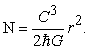(1.1)

The total energy formula of a black hole is (1.2). r is the radius of a black hole.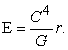(1.2)

The entropy formula of a black hole is (1.3). r is the radius of a black hole. n is the degrees of freedom of the particles that make up the black hole.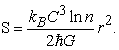(1.3)

The temperature formula of a black hole is (1.4). r is the radius of a black hole. n is the degrees of freedom of the particles that make up the black hole.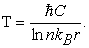(1.4)

Here, the author corrects two errors in the previous paper and apologizes to the reader.

To the black hole merging model in the previous paper stated 2, there were inaccuracies and uncertainties. It's not two black holes that merge together. The correct statement is that. The center is a black hole, and the other object merging into the black hole is actually a normal particle. The mass of the particle is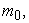which meets the condition of (5.1) in the previous paper 2. Repeat this process, so it can be concluded that the most central particle is the original seed of the black hole. The particles system, which these particles combine to form, as a whole, for the outside, is a black hole. Entering the inside of this black hole, these particles inside the black hole are not black holes, just normal particles. In fact, this merged black holes is a composite object. This kind of composite black hole is a thermodynamic system, so it has entropy and temperature. These particles that form the black hole meet the condition (5.1). This particular physical system, as a whole, for the outside, is a system with the property of black hole.

In the projection gravitation theory, every particle with rest mass has the property of black hole radius,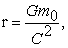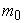is the rest mass of a particle. It is important to distinguish precisely the similarities and differences between concepts. Particle with rest mass ofin the merging process has an inherent length property of black hole radius, but the particle is not a real black hole.

So, in projection gravitation, a black hole is actually a particles system. This particles system, as a whole, for the outside, manifests itself as a black hole. However, the individual particles that make up this black hole are not black holes in fact. There are great differences between the concept of black holes in projection gravitation, the concept of black holes in other gravitation theory. Please note the difference here.

In the previous paper 2, author calculated that the black hole temperature formula (5.10) was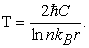There is a calculation error here. The correct result isThis composite black hole model in projection gravitation is a physical system in the thermodynamic sense, with internal structure, internal distribution, and is a true thermodynamic black hole. The entropy of this kind of black holes has a reasonable origin of physics.

In the discussion of the merging process of black hole formation, author obtains the merging condition (1.5). This condition is based on the quantum mechanical equation of the gravitation field. Only particles that meet this condition can be combined to form a system of black holes.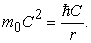(1.5)

The specific physical significance of the condition (1.5) was not carefully elaborated in the previous paper 2. So, in this paper, authors continue to discuss this merging process of black hole formation.

In the merging process of black hole, there should be a special particle at the center of the black hole. The central particle, which is the original seed of the black hole. This primitive seed attracts another particle close to it. Another particle satisfies the condition (1.5). The two particles merge together to form system of a black holes. In this way, the black hole is growing, forming a large black hole.

For ease of description, use a new constant symbol D to identify the constant of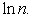Please pay attention to it.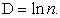(1.6)

From (1.1), (1.2) and (1.4), we can get the formula (1.7).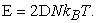(1.7)

It is not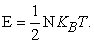Why there are differences in constants, this topic needs further study.

In fact, to (1.5), the more general case would be as (1.8). The numerical change of constant value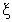only changes the value of the constant in the derivation result and does not change the physical essence of the theory. The exact value of the constant valueneeds to solve the exact solution of the equation of the projection gravitation field wave function in order to be determined. Also, there may be different types of particles, and thevalues of the particles may be different.

### 3. Temperature Condition in Merging Process

As shown in Figure 1, there have two objects. One is A, and it is a black hole. Its rest mass is M. r is its radius of black hole,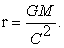The other is B, rest mass is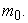The two objects merge together to form a new black hole.

According to the merging model of black hole formation in the previous paper 2, particle B must meet the condition, the formula (2.1).(2.1)

What is the physical significance of this formula? This formula means that. If there is a black hole A whose black hole radius is r. If there is another particle B, can merge with A to form a new black hole. The rest mass of the particle B must meet the formula (2.1). Particles with other rest mass cannot be combined with particle A to form a new black hole. This is the merging condition of black hole formation.

Condition (2.1) is derived out from the quantum mechanical wave function equation of projection gravitation. Only if this condition is met in the gravitation field of A, the particle B has the lowest energy, so it has possible to merge with A. This condition, from the point of view of quantum mechanics, is an obvious condition. Two particles are merged, and it is clear that the two particles should have the greatest chance of getting close to each other, and the most possible to merge. B is at the lowest energy level in the A gravitation field, the probability of being close to A is the greatest, and the probability of a merger occurring is the greatest. This is a very obvious result. From the quantum energy formula of projection gravitation, it can be seen that when particle B is at the lowest energy in A gravitation field, the rest mass of B meets the condition (2.1). This is the physical significance of (2.1) in quantum mechanics. This condition is actually the result of the calculation of quantum mechanical equations in projection gravitation. (2.1) is an energy condition.

Please note that in the projection gravitation, a black hole is an empty hole in fact, and the radius of the black hole is actually an empty hole radius. The essence of the merge process is that two objects are close to each other. Because object A has the empty hole radius r, so the minimum distance between B and A is r. So the condition (2.1) is actually a distance condition also. According to quantum mechanics, if the minimum distance can be reached, it can only be that particle B is at the lowest energy.

In thermodynamics, the temperature of particle B satisfies (2.2).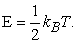(2.2)

So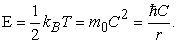So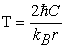(2.3)

(2.3) is the temperature of particle B.

The temperature of the black hole A is (1.4).

The temperature of particle B, and the temperature of black hole A, has only one difference in proportional constant. About go constant, roughly speaking, can be considered as temperature equality.

Therefore, from the thermodynamic point of view, the merging process of black hole formation, there is a temperature condition. Two objects must meet this temperature condition in order to merge to form a black hole. Two objects that do not meet this temperature condition cannot merge to form a black hole.

Condition (2.1) is equivalent to a temperature condition.

It can also be described in such a way to the merging process of the formation of black hole. Particle B, incorporated into a black hole A, forms a new black hole. Particle B must meet this temperature condition in order to fit into black hole A. Otherwise, particle B cannot be integrated into black hole A. Therefore, for a black hole, only external particles that meet this special temperature condition can be integrated into the black hole.

From the quantum mechanical wave function equation of projection gravitation, there is an energy condition in the process of merging. From the thermodynamics, there is a temperature condition in the process of merging. There is a wonderful correlation between the two. This may be the physical origin of gravity with a wonderful thermodynamic nature.

We approximately think it. The particle momentum is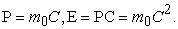Particle is close to the black hole, and the minimum distance is the black hole radius r. According to the principle of uncertainty, approximately is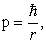so getIt is the condition (2.1). So, according to the principle of uncertainty, can derive out the condition (2.1). (2.1) is equivalent to a temperature condition. So, we can think so. In quantum gravitation, there is a wonderful correlation between the uncertainty principle and the temperature. Temperature is correlated with entropy. Entropy and uncertainty are related. The principle of uncertainty is a sign of uncertainty. Therefore, the author boldly speculates that the uncertainty principle may be the real physical source of entropy. This topic is worthy of further study.

Let's look at the property of particle B from a temperature perspective. Particle B satisfies the formula (2.2). Obviously, particle B is not a black hole. According to the merge condition (2.1), the larger r, the smaller the energy of particle B. According to (2.2), the lower the particle temperature. However, if particle B is a black hole, the smaller the particle energy, the smaller the radius of the black hole, the higher the temperature of the black hole. The two are quite the opposite. So, from here, it can also be seen that particle B, which merges into black hole A, is not a black hole, just a normal particle. A large number of particles that meet the merger condition (2.1) form a particle system. As a whole, the particle system exhibits the property of a black hole. A black hole is just a special collection of particles, a special particle system. A black hole is not a single physical object, but a system of particles collection. This particles system, as a whole, exhibits the property of a black hole, has entropy and has temperature.

The merged black hole, the total energy of its particles meets the formula (1.2). Total energy can be converted to total mass, so get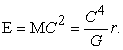So get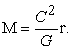So, the radius of the black hole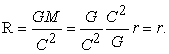The radius of the merged black hole is indeed equal to the total radius of the particle system. So this merged particles system, as a whole, is indeed a black hole. So, in projection gravitation, a black hole is just a special type of particle collection object.

We can also find that the coefficientchange in (1.8) only changes the proportional value between the temperature of the two objects. It does not change the essence of a fixed proportional relationship between the temperature of the two objects.

### 4. Derive out Verlinde’s Entropy Gravity Proposal Formula

Continuing the discussion above, in this merging process, let's calculate the entropy of particle B.

To particle B, it satisfies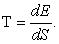So get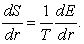(3.1)

Because the energy of particle B satisfies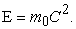And it satisfies (2.1). So get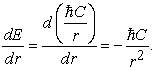(3.2)

In merging process, particle B satisfies the temperature condition. So the temperature of particle B is (3.3).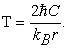(3.3)

Take (3.2) and (3.3) into (3.1), so get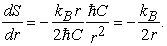(3.4)

From (2.1), can get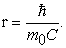Take it into (3.4), so get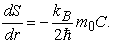(3.5)

This is the change of the entropy of particle B during the merging process. The negative sign indicates the direction of the gravitational pull of particle B. The change of entropy is caused by gravitation. Therefore, this change of entropy reflects the property of the gravitational pull of particle B.

As you can see, in addition to the differences in constants, the form of the formula (3.5) is exactly the same as Verlinde's entropy gravity proposal formula.

The key of the whole derivation process lies in the existence of temperature condition (2.1). So, as you can see from here, Verlinde's entropy gravity proposal formula is actually a result in the process which particle merge into a black hole. Verlinde's entropy gravity proposal formula can be derived out in the merging process of black hole formation. So Verlinde's entropy gravity proposal formula is just an export of projection gravitation. So Verlinde's entropy gravity proposal formula is equivalent to the temperature condition (2.1).

In fact, the theory of entropy gravity hypothesis is very similar to the merging process of the formation of black holes in projection gravitation. The gravity of entropy is a particle incorporated into a virtual holographic screen, the radius of the holographic screen increases. In projection gravitation it is a merging of particles and a black hole to form a new black hole, with an increased radius of the black hole.

But in fact, in the projection gravitation, particles have always been particles, and black holes have always been black holes. just two objects close together, from the outside, it seems that the formation of a new black hole. The radius of the black hole is increasing. Merging just reduce the distance, and not really blend in. Integration is only an observation from an outside perspective.

In this process of merging, it can be seen that only particles that meet the condition (2.1) can be integrated into the black hole. Other particles cannot be integrated into a black hole. But the theory of entropy gravity hypothesis assumes that all particles can be integrated into a holographic screen. Therefore, from the derivation in projection gravitation, the accuracy of the assumption of entropy gravity, there is doubt, it is questionable. The Verlinde’s entropy gravity proposal theory is not universally correct, but only in special circumstances.

From the discussion of the merger process above, it can be seen that the holographic principle and the entropy of black holes in projection gravitation are exactly corresponding. Holographic screens can be thought of as virtual black holes. In projection gravitation, a black hole is actually an empty hole, and not a single object, but a system of particles. This particle system, on the whole, for the outside world, shows the property of a black hole, with black hole entropy, with black hole temperature. In the General Relativity, a black hole is a bottomless abyss of that inhales all matter. But in the projection gravitation, it's not like this. The empty hole model in the projection gravitation is actually more in line with the concept of holographic screen.

### 5. Derive out Unruh Formula

Let’s continue to discuss the merging process.

The temperature of particle B is (2.3).

To the black hole A, it satisfies(4.1)

Take (4.1) into (2.3), get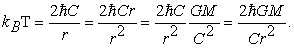(4.2)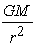is the gravitational acceleration on the surface of the black hole A. So get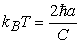(4.3)

In addition to the differences in constants, (4.3) is exactly the same as Unruh formula.

So, we derive out the results of the Unruh formula from the process of merging. Therefore, it can be seen that the Unruh formula is only a manifestation of the property of this merging process.

The Unruh formula can also be derived out directly from the black hole entropy. Therefore, it can be concluded that the Unruh formula is just another expression of the black hole entropy formula.

The temperature of the black hole is (1.4), so get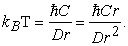(4.4)

Because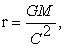so get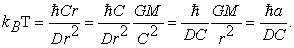(4.5)

It is only one constant difference from (4.3).

Therefore, we can see that the Verlinde's entropy gravity proposal formula and Unruh formula are the property of merging process of the black hole formation. The two are actually equivalent. Gravitation can no longer be derived out using the Verlinde's entropy gravity proposal formula and the Unruh formula. Verlinde's entropy gravity proposal is circular reasoning in fact.

The gravitational acceleration on the surface of the black hole,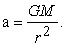Andso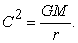So we can get a very simple result.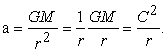(4.6)

The gravitational acceleration on the surface of a black hole has a very simple relationship between the radius of the black hole. (4.6) and (1.4) take very similar forms. There is only a constant difference between the two. Therefore, it is clear that the black hole temperature and the surface acceleration of the black hole have a simple proportional relationship. It is actually a result derived from the projection gravitation theory.

The simplicity of formula (4.6) is surprising. To an object, its gravitational acceleration is not infinitely large and has a maximum value. This seems to be contrary to our conventional understanding of gravity, such as Newton's gravity, such as General Relativity. For Newton's gravity, r can be infinitely small, and gravitational acceleration can be infinitely large. For General Relativity, particles can be infinitely close to the radius of a black hole, time can be completely stagnant, and equivalent gravitational acceleration can be infinitely large. But, in projection gravitation, because the black hole is an empty hole in fact, so the distance has a minimum value, so the its gravitational acceleration has a maximum value.

### 6. Conclusion

In the above discussion, the author discusses in detail the merging process of black hole formation in projection gravitation theory. The surprising results are derived out. In the merging process, we derive out the Verlinde's entropy gravity proposal formula, and we derive out the Unruh formula. It is also found that the Verlinde's entropy gravity proposal formula and the Unruh formula are actually equivalent, both of which are the same physical source, both from the merging process which form the black hole. The Verlinde’s entropy gravity proposal theory is not universally correct, but only in special circumstances.

To the quantum mechanical equations of projection gravity field, there is a curious correlation between the particle energy and the thermodynamic temperature, between the uncertainty principle and the thermodynamic temperature. Why gravitation has a very special thermodynamic property? This association is the physical origin. This topic is well worth studying.

The projection gravitation theory can derive out many meaningful physical results. It is a reasonable quantum gravitation theory, and has important research value. The authors expect more researchers to join the study of projection gravitation theory, revealing more properties of gravitation.

### References

  Xiao Lin Li. Projection Gravitation, a Projection Force from 5 dimensional Space-time into 4-dimensional Space-time International Journal of Physics, 2017, 5(5), 181-196. In article  XiaoLin Li. Empty Hole Entropy and Black Hole Entropy in Gravitation International Journal of Physics, 2018, 6(5), 155-160. In article View Article  Bekenstein,Jacob D. (April 1973). Black holes and entropy Physical Review D 7(8): 2333-2346. In article View Article  Bardeen,J. M.; Carter, B.; Hawking, S. W. The four laws of black hole mechanics Comm.Math. Phys. 31 (1973), no. 2, 161-170. In article View Article  E. P. Verlinde. On the Origin of Gravity and the Laws of Newton JHEP 1104 (2011) 029 [arXiv:1001.0785 [hep-th]]. In article View Article  G.’t Hooft. Dimensional reduction in quantum gravity ar Xiv:gr-qc/9310026. In article  W. G. Unruh. Notes on black hole evaporation Phys. Rev. D 14, 870 (1976). In article View Article  David Chandler introduction to modern statistical mechanics ISBN-13: 978-0195042771. In article  R. P. Feynman, R. B. Leighton, M. Sands. The Feynman Lectures on Physics (Volume I,II,III). ISBN 9787506272476, ISBN 9787506272483, ISBN 9787506272490. In articleThis work is licensed under a Creative Commons Attribution 4.0 International License. To view a copy of this license, visit http://creativecommons.org/licenses/by/4.0/